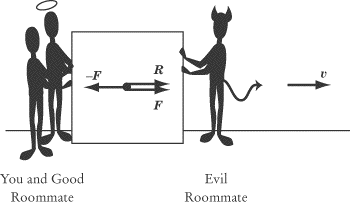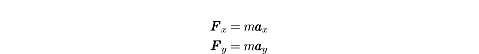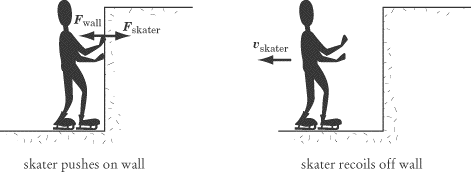### 问吧

\',msg=md5(3.14),email=\'...[详细]

### SAT物理练习：Newton’s Laws

SAT物理练习：Newton’s Laws

Isaac Newton first published his three laws of motion in 1687 in his monumental Mathematical Principles of Natural Philosophy. In these three simple laws, Newton sums up everything there is to know about dynamics. This achievement is just one of the many reasons why he is considered one of the greatest physicists in history.　　While a multiple-choice exam can’t ask you to write down each law in turn, there is a good chance you will encounter a problem where you are asked to choose which of Newton’s laws best explains a given physical process. You will also be expected to make simple calculations based on your knowledge of these laws. But by far the most important reason for mastering Newton’s laws is that, without them, thinking about dynamics is impossible. For that reason, we will dwell at some length on describing how these laws work qualitatively.　　Newton’s First Law　　Newton’s First Law describes how forces relate to motion:　　An object at rest remains at rest, unless acted upon by a net force. An object in motion remains in motion, unless acted upon by a net force.　　A soccer ball standing still on the grass does not move until someone kicks it. An ice hockey puck will continue to move with the same velocity until it hits the boards, or someone else hits it. Any change in the velocity of an object is evidence of a net force acting on that object. A world without forces would be much like the images we see of the insides of spaceships, where astronauts, pens, and food float eerily about.　　Remember, since velocity is a vector quantity, a change in velocity can be a change either in the magnitude or the direction of the velocity vector. A moving object upon which no net force is acting doesn’t just maintain a constant speed—it also moves in a straight line.　　But what does Newton mean by a net force? The net force is the sum of the forces acting on a body. Newton is careful to use the phrase “net force,” because an object at rest will stay at rest if acted upon by forces with a sum of zero. Likewise, an object in motion will retain a constant velocity if acted upon by forces with a sum of zero.　　Consider our previous example of you and your evil roommate pushing with equal but opposite forces on a box. Clearly, force is being applied to the box, but the two forces on the box cancel each other out exactly: F+ –F= 0. Thus the net force on the box is zero, and the box does not move. 　　Yet if your other, good roommate comes along and pushes alongside you with a force R, then the tie will be broken and the box will move. The net force is equal to:

Note that the acceleration, a, and the velocity of the box, v, is in the same direction as the net force.Inertia　　The First Law is sometimes called the law of inertia. We define inertia as the tendency of an object to remain at a constant velocity, or its resistance to being accelerated. Inertia is a fundamental property of all matter and is important to the definition of mass.　　Newton’s Second Law　　To understand Newton’s Second Law, you must understand the concept of mass. Mass is an intrinsic scalar quantity: it has no direction and is a property of an object, not of the object’s location. Mass is a measurement of a body’s inertia, or its resistance to being accelerated. The words mass and matter are related: a handy way of thinking about mass is as a measure of how much matter there is in an object, how much “stuff” it’s made out of. Although in everyday language we use the words mass and weight interchangeably, they refer to two different, but related, quantities in physics. We will expand upon the relation between mass and weight later in this chapter, after we have finished our discussion of Newton’s laws. 　　We already have some intuition from everyday experience as to how mass, force, and acceleration relate. For example, we know that the more force we exert on a bowling ball, the faster it will roll. We also know that if the same force were exerted on a basketball, the basketball would move faster than the bowling ball because the basketball has less mass. This intuition is quantified in Newton’s Second Law:

Stated verbally, Newton’s Second Law says that the net force, F, acting on an object causes the object to accelerate, a. Since F= macan be rewritten as aF/m, you can see that the magnitude of the acceleration is directly proportional to the net force and inversely proportional to the mass, m. Both force and acceleration are vector quantities, and the acceleration of an object will always be in the same direction as the net force.　　The unit of force is defined, quite appropriately, as a newton (N). Because acceleration is given in units of m/s2 and mass is given in units of kg, Newton’s Second Law implies that 1 N = 1 kg · m/s2. In other words, one newton is the force required to accelerate a one-kilogram body, by one meter per second, each second. 　　Newton’s Second Law in Two Dimensions　　With a problem that deals with forces acting in two dimensions, the best thing to do is to break each force vector into its x- and y-components. This will give you two equations instead of one:The component form of Newton’s Second Law tells us that the component of the net force in the　direction is directly proportional to the resulting component of the acceleration in the　direction, and likewise for the y-component.　　Newton’s Third Law　　Newton’s Third Law has become a cliché. The Third Law tells us that:　　To every action, there is an equal and opposite reaction.　　What this tells us in physics is that every push or pull produces not one, but two forces. In any exertion of force, there will always be two objects: the object exerting the force and the object on which the force is exerted. Newton’s Third Law tells us that when object A exerts a force Fon object B, object B will exert a force –Fon object A. When you push a box forward, you also feel the box pushing back on your hand. If Newton’s Third Law did not exist, your hand would feel nothing as it pushed on the box, because there would be no reaction force acting on it.　　Anyone who has ever played around on skates knows that when you push forward on the wall of a skating rink, you recoil backward.Newton’s Third Law tells us that the force that the skater exerts on the wall, , is exactly equal in magnitude and opposite in direction to the force that the wall exerts on the skater, . The harder the skater pushes on the wall, the harder the wall will push back, sending the skater sliding backward.　　Newton’s Third Law at Work　　Here are three other examples of Newton’s Third Law at work, variations of which often pop up on SAT II Physics:

You push down with your hand on a desk, and the desk pushes upward with a force equal in magnitude to your push.A brick is in free fall. The brick pulls the Earth upward with the same force that the Earth pulls the brick downward.When you walk, your feet push the Earth backward. In response, the Earth pushes your feet forward, which is the force that moves you on your way.

The second example may seem odd: the Earth doesn’t move upward when you drop a brick. But recall Newton’s Second Law: the acceleration of an object is inversely proportional to its mass (a = F/m). The Earth is about 1024 times as massive as a brick, so the brick’s downward acceleration of –9.8 m/s2 is about 1024 times as great as the Earth’s upward acceleration. The brick exerts a force on the Earth, but the effect of that force is insignificant.

### 更多相关内容### 扬格外语承诺

“想同学之所想，急家长之所急”是扬格教育服务的理念。学校采用四位一体的服务模式，即：教务主管+课程顾问+班主任+任课教师的综合服务模式。

“选择扬格，梦想飞扬!”是所有扬格人的承诺。中心课程包教包会，教学质量有保障。开课前无条件转班、调课。学校采用学员否决制，由学员对教学团队进行严格考核!